# How to Add a Variance to a Chart

Add a variance to your chart to show the difference between versions of a measure or to show the difference between time periods.

## Context

You can create a variance for any two measures, even if the measures do not appear in your base chart. You can create variances that don't include the versions for the measures, as well as variances that do include time periods.

## Procedure

1. Add a variance in one of the following ways:
• From a canvas page:
1. Select your chart, open the Designer, and then select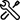(Builder).

2. In the Variance area, select Add Variance.

Tip
If you cannot see a variance area, find Chart Structure and select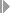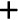(Add Chart Components)Add Variance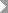.
• From a grid page:
1. In the table, select some data.

2. Open the Examine panel, select(more), and then selectVarianceAdd Variance.

2. Choose specific measures or All Measures in Use (Dynamic).
3. Choose the versions or time periods.
4. From the Display Options area, apply the following options:
Option Description
Name Use the default name or provide a different name for the variance.
Invert Colors Reverse the color scheme from the following default values: a positive variance is shown in green, and a negative variance is shown in red.
Set No Data as Zero To include a NULL data point in your variance, set it to the value zero.
Scale with Base Chart Default setting keeps the variance chart scale the same as the chart scale. If you want the variance to have its own scale, clear the check box.
Show Difference as

Choose whether you want to display the variance as Numeric, as a Percentage, or both.

When you choose Percentage you can also choose to use an Absolute Base Value. Absolute values are useful if you are comparing negative values, but have a positive change (For example, (-20) - (-40) = +20. The percentage change (+20/-40) would be -50% if you did not use absolute values.)

View Variance as
• Bar – displays a classic variance chart beside the regular chart.

• Data Label – adds variance information to the data labels.

Use data labels if there is no room to add a variance chart, or if you want variance information for charts that cannot use a variance chart.

• Integrated – displays the variance data as an overlay on the bar chart.

5. When finished, select OK.

## Results

A variance chart or variance information is added to your visualization. The variance bars are color-coded based on the account type and whether an increase is desirable or not. For example, an increase in income would be welcome (green), but an increase in expenses would not (red).

Variance Bar Color Based on Account Type

Account Type

Decrease (-)

Increase (+)

INC – Income

Red

Green

EXP – Expense

Green

Red

AST – Assets

Red

Green

LEQ – Liabilities & Equities

Green

Red

Tip
To hide the variance label, select(More Actions)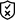(Show / Hide)Variance Label.

## Next Steps

1. Open the Designer and select(Builder).
2. In the Variance area, find the variance calculation and select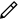Edit.
1. Open the Examine panel, select(more), and then selectVarianceEdit Variance.